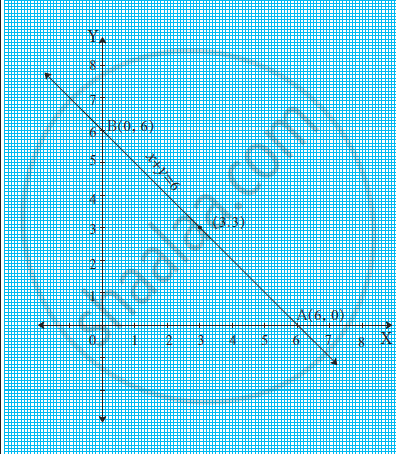Advertisement Remove all ads

# Draw the Graph of X + Y = 6 Which Intersects the X-axis and the Y-axis at a and B Respectively. Find the Length of Seg Ab. Also, Find the Area of δ Aob Where Point O is the Origin. - Algebra

Advertisement Remove all ads
Advertisement Remove all ads
Advertisement Remove all ads

Draw the graph of x + y = 6 which intersects the X-axis and the Y-axis at A and B respectively. Find the length of seg AB. Also, find the area of Δ AOB where point O is the origin.

Advertisement Remove all ads

#### Solution

(a) Draw the graph of x + y = 6

x + y = 6

 x 6 3 0 y 0 3 6 (x, y) (6, 0) (3, 3) (0, 6)(b) In Δ AOB, by Pythagoras theorem,
AB2 = OB2 + OA2 = 62 + 62 = 2 × 36
∴ AB = 6sqrt(2)
OR, A(6, 0) and B(0, 6)
∴ d(A, B) = sqrt((x_2-x_1)^2 + (y_2-y_1)^2)

= sqrt((0-6)^2 + (6-0)^2) = sqrt((36+36) ) = sqrt(72) = 6sqrt(2)
A(Δ AOB) = 1/2 × product of sides making right angle
= 1/2 × 6 × 6 = 18 sq. unit

Concept: Graphical Method of Solution of a Pair of Linear Equations
Is there an error in this question or solution?
Advertisement Remove all ads
Share
Notifications

View all notifications

Forgot password?